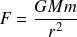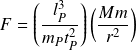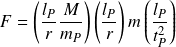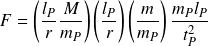# Gravitational force

Gravitational force characterizes the strength of a gravitational field. The formula quantifies the gravitational field in terms of the force it exerts on a second body.

Gravitational force potential is determined by the mass density and distance operators acting on the maximum force potential. The formula also uses the rest mass operator to quantify the mass of a second body in relation to the Planck mass. The elementary formula stated in operators and potentials is

## Historical formula

The elementary formula can be derived from the historical formula for gravitational force which uses the gravitational constant. The historical form of the equationcan be re-stated in elementary form by replacing the gravitational constant with natural units of length, mass, and time. The formulacan then be grouped into operators and potentialsInserting a hidden quantity of Planck mass in the numerator and denominator states the formula in terms of three operators acting on the maximum Planck force potential.The following illustration shows how the historical formula transforms into elementary form.

Gravitational force: conversion from historical to natural formula

Gravitational force